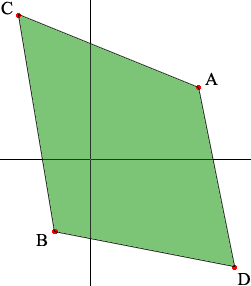SEARCH HOMEMath Central Quandaries & QueriesQuestion from sefora, a student: The coordinates of the vertices of quadrilaterals are given. Draw each quadrilateral on a grid. Determine whether it is a rectangle (show your work) P(5,1), Q(-4,4), R(-6,-2), S(3,-5)Sefora,

Let me try 4 different points

A(3,2), B(-1,-2), C(-2,4), D(4,-3)

Here is my sketchI plotted the four points and them to form 4 line segments with no internal crossings. It sure doesn't look like a rectangle. If it were a rectangle then the adjacent sides BD and DA would be perpendicular. I'll check by calculating the slopes

Slope of BD: [-3 - (-2)]/[4 - (-1)] = -1/5
Slope of DA: [2 - (-3)]/[3 - 4] = 5/-1 = -5

If these line segments were perpendicular then slope DA = -1/[Slope BD], but this is not true so ACBD is not a rectangle.

I hope this helps,
PennyMath Central is supported by the University of Regina and The Pacific Institute for the Mathematical Sciences.